Next: D1.1.1 Segment and Device Up: D1. Iteration Blocks Previous: D1. Iteration Blocks

## D1.1 Equations and Quantities

The user can select which equation set is to be solved. To allow for a more flexible equation assembly, the equations are split into their constituent terms which can be selected independently. This allows for implementing iteration schemes as reported in  where, e.g., the recombination terms in the continuity equations or the contribution of the carrier densities to the charge in Poisson's equation are neglected.

When used for the first time, for each equation the appropriate quantities are automatically created and initialized with proper initial values. These initial values are either taken from a previous simulation or are calculated to give reasonable defaults as follows.

• The potential is initialized with the built-in potential (cf. equation (6.9)).
• The initial carrier concentration is calculated using a charge neutrality assumption.
• The lattice temperature is set to the average of the contact temperatures.
• The carrier temperatures are set equal to the lattice temperature.
The quantities are added to the solution vector and remain there until the end of the simulation. Each quantity can be separately marked for solving in the next Newton iteration. Only the equations associated with the marked quantities will be used to build up the equation system and of course only the marked quantities will be updated after solving the equation system. However, all quantities already available in the solution vector can be used to calculate the system matrix. The available equations are summarized in the following. A more detailed description of the equations can be found in Chapter 3. The equations are given in differential form, whereas they are implemented in integral form using the box integration scheme .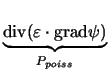=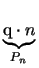-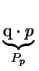-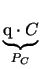-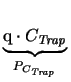(D1)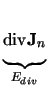=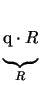+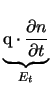(D2)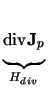=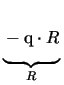-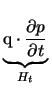(D3)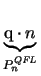= q . NC . exp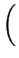-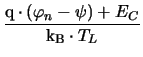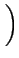(D4)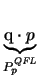= q . NV . exp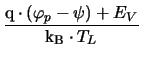(D5)=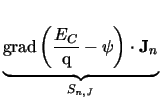-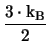.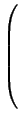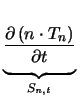+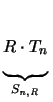+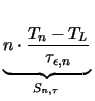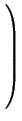(D6)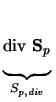=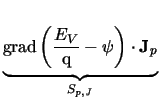-.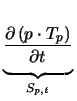+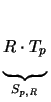+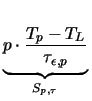(D7)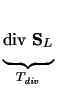=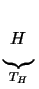-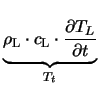(D8)

The unknown quantities of this equation system are the electrostatic potential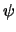, the electron and hole concentrations n and p, the carrier temperatures Tn and Tp, and the lattice temperature TL. C denotes the net concentration of the ionized dopants, CTrap denotes the concentration of the occupied traps,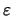is the dielectric permittivity of the semiconductor, and R is the net recombination rate. Since only a few combination of terms yield meaningful results, these terms are collected to so-called model groups to simplify the selection mechanism.

Currently two different versions of Ediv exist depending on the current model used ((3.5) or (3.7)), namely EdivDD and EdivHD, vice-versa for holes. The groups resulting from the equation assembly models given above are listed in Table D.1.

 Group name Equation assembly models electrons EdivDD, Pn electronsTrans Et electronsQFL PnQFL electronsHD EdivHD, Pn, Sn, div, Sn, J, Sn,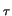electronsHDTrans Et, Sn, t holes HdivDD, Ph holesTrans Ht holesQFL PpQFL holesHD HdivHD, Pp, Sp, div, Sp, J, Sp,holesHDTrans Ht, Sp, t potential Ppoiss, PC R,, PCTrap, Sn, R, Sp, R selfHeating Tdiv, TH selfHeatingTrans Tt

The important recombination groups are written as <recombination>, denoting that several different mechanisms exist which split the terms given above into terms handling only one single effect. Currently available are Shockley-Read-Hall recombination, Auger recombination, direct recombination, band to band tunneling, and impact ionization. In addition, interface groups are available which handle the interface and boundary conditions. These groups can be considered to be complete in the following sense: if it is desired to simulate the Poisson equation, e.g., inside an oxide, only the potential group is necessary. This is done by the following iteration block:

Iterate
{
Scheme
{
models = "potential,potentialInt";
...
}
}


If one is also interested in the electron concentration, e.g., in the channel of a HEMT, the electron group has to be added:

Iterate
{
Scheme
{
models = "potential,potentialInt,electrons,electronsInt";
...
}
}


If the derivative of the electron concentration with respect to time is relevant, e.g., for a transient simulation, the electronTrans group has to be added:

Iterate
{
Scheme
{
models = "potential,potentialInt,"
"electrons,electronsInt,electronsTrans";
...
}
}


 Group Name Created Quantity Classes electrons Potential ElectronConcentration electronsTrans ElectronConcentration electronsQFL Potential ElectronConcentration electronsHD Potential ElectronConcentration ElectronTemperature electronsHDTrans ElectronConcentration holes Potential HoleConcentration holesTrans HoleConcentration holesQFL Potential HoleConcentration holesHD Potential HoleConcentration HoleTemperature holesHDTrans HoleConcentration potential Potential Potential ElectronConcentration HoleConcentration selfHeating LatticeTemperature selfHeatingTrans LatticeTemperature potentialInt Potential on both segments electronsInt Potential on both segments ElectronConcentration on both segments electronsHDInt Potential on both segments ElectronConcentration on both segments ElectronTemperature on both segments terminal ContactVoltage ContactCurrent ContactCharge ContactTemperature BoundaryCurrent BoundaryElectronCurrent BoundaryHoleCurrent BoundaryConductionCurrent BoundaryDisplacementCurrent

Several tests are implemented to simplify model assembly. Among these test are the following:
• Each group has a list of material classes for which it can be used, e.g., the electrons group will not be used for materials of class Insulator.
• The carrier concentrations are controlled either by one of the transport equations or by the QFL approximation. So the groups electrons, electronsHD, and electronsQFL are implemented to be mutually exclusive. E.g., if one of the transport equations is available, the QFL model is automatically disabled. This is done without a warning to reduce the number of if statements in the input deck.
• From (D.2) and (D.3) it follows that recombination and generation modeling is only consistent with the rest of the equation system when both carrier types are properly modeled by transport equations. Hence all recombination models check for the existence of the two continuity equations. Again, in case this check fails, no warning is issued, the model is simply disabled.
• The interface and contact models automatically check for a proper material class combination in order to become active. E.g., the carrier contact model checks for an ideal-conductor semiconductor interface. Hence the interface groups can be used for all boundaries without worrying about further details.

As pointed out above, each group automatically creates the quantities which form the unknowns of the equation system. Each quantity belongs to a so-called quantity class, e.g., the potentials inside all insulator, conductor, and semiconductor segments belong to the quantity class Potential. Table D.2 lists the quantity classes and the model groups which create them. It is important to note that each quantity is created and initialized when it is first requested by one of the model groups. The mere creation of a quantity does not imply that there is a controlling equation available. In case the user registers only the electrons group on one segment, the quantitiesand n are created. The potentialis initialized to the built-in potential whereas the electron concentration n is computed using a charge-neutrality assumption. As there has been no Poisson equation requested (group Potential has not been used), the electron continuity equation will be solved stand-alone.

The same is true for interface models. When using for instance the HD interface group, carrier temperature quantities on both sides of the interface are created and are initialized to the lattice temperature. However, by simply creating these quantities the interface model does not make any assumptions about the transport models used on either side. It is possible to use a DD model on one side and a HD model on the other side which implies an equilibrium boundary condition for the carrier temperatures as the carrier temperatures are set equal to the lattice temperature in this case.

The terminal group needs some further attention. It creates an abundance of quantities which might seem unnecessary at a first glance. The quantities whose names start with Contact are the terminal quantities which are used to formulate the boundary conditions. The quantities with names beginning with Boundary are supplementary quantities which represent the current components and are used to simplify calculation of the contact current.

For mixed-mode simulations the basic semiconductor equations are augmented by the circuit equations resulting from the modified nodal approach (MNA) and the MNA equations for the thermal equivalent circuit. It is worthwhile to note that for the KCL equation the node voltage vector is split into two quantity classes namely node voltages (NodeVoltage) and fixed node voltages (FixNodeVoltage), respectively. Since each quantity class can be marked separately for the solving process, this separation has the major advantage that the fixed node voltage class can be arbitrarily solved or not. One way to utilize this feature is to emulate the behavior of typical circuit simulators like SPICE. To aid convergence, SPICE allows the user to set some critical node voltages to a constant value, iterate until convergence, release the critical node voltages, and continue iteration until final convergence. This has been implemented in the MixedDD and MixedHD iteration schemes.

Schemes
{
MixedDD
{
DDPre : DDBlock
{
ignoreQuantities = "FixNodeVoltage";
}
DDPre : DDBlock;
}
}

Iterate
{
Scheme : ~Schemes.MixedDD;
}


However, due to the generality of this concept, it is possible to design own iteration schemes with a different behavior. One useful possibility might be to fix some node voltages only at distinct time points to their initial or last value (which is internally the same).Next: D1.1.1 Segment and Device Up: D1. Iteration Blocks Previous: D1. Iteration Blocks
Tibor Grasser
1999-05-31